Some quadratic expressions factor easily and some don’t.

If a quadratic expression factors easily, then we use the factoring process to locateintercepts of the parabola and we use it to solve quadratic equations in general.

If a quadratic expression doesn’t factor easily then it is possible that either the parabola doesn’t cut theaxis at all, or that theintercepts are irrational. In that case, we use a different method to find theintercepts (or solutions to the equation).

# Review

The general form for a quadratic expression isWhen the coefficient of,we have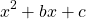. These expressions are quite straightforward to factor.

## Example 1:We need to find numbersandsuch thatA little thought leads us to the valuesand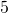becauseand.

Therefore we haveCheck this answer by expanding the brackets with FOIL or otherwise to confirm that the expression on the left hand side is equivalent to the expression on the right hand side.

## Example 2We need to find numbersandsuch thatThe valuesandwork here becauseand.

Therefore we have## Example 3: Difference of two squares

Check that this works by multiplying out the brackets:In generalOr, to be even more generalDifference of two squares questions

1.2.3.# When the coefficient a is not 1: simpler problems

These can also be quite straightforward if there turns out to be a common factor in all three terms, or if it can be understood as the difference between two squares, or if there is no constant term andis a common factor.

Example 4: No constant termThe highest common factor of these two terms is.Example 5: There is a common factor between all three termsFirst we notice that 2 divides all three terms. Let’s factor it out:Now, within the brackets, we have a trinomial withand so we simply find a factor pair that multiplies to 4 and adds to -5. I’m thinking -4 and -1 will do it!# When the coefficient a is not 1: more complicated

If there is no common factor and all three terms are present, factoring can be quite challenging.

Here are four methods:

# Factoring by Elimination

First, remember that factoring is about efficiency. If some time has been spent and no factors have been found, we may use an alternative technique (graphing, quadratic formula, completing the square…).

Here is how to do it by elimination, that is, by testing the limited number of possibilities until a solution is found:

## Example 6:We need to examine the factor pairs of 3 and of 20:  (1,3) is the only factor pair of 3. Factor pairs of 20 include (1,20); (2,10); (4,5)

We can beginas (3,1) is the only factor pair of 3. Considering FOIL, the ‘F’ has now been dealt with. As we are only going to insert a factor pair of 20 the ‘L’ will also be dealt with.

We only need to consider the ‘O’ and the ‘I’. These must add to -11. We have three factor pairs to consider, knowing that one of the pairs must be negative to yieldas the last term.

Let’s try the factor pair (1,20) in all configurations. We require the middle term to be.Let’s try the factor pair (2, 10) in all configurations but this time, just consider the O and the I terms, and we need not swap the signs as both give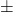the same value: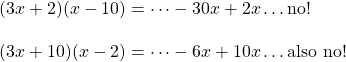Now the factor pair (4,5):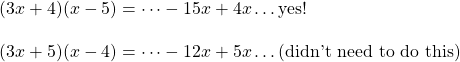Therefore.

It is good to remember that the number of possible factor pairs is generally quite limited, which makes this method reasonably quick.

Alternatively – here’s Mario’s Math describing ‘decomposition’, or ‘splitting the middle term’.

# Practice finding x-intercepts (roots)

As we saw on the page Graph a Parabola, the factored form can lead us quickly to the-intercepts of a parabola. To findintercepts we set. In this applet the quadratic functions can be written in factored form.